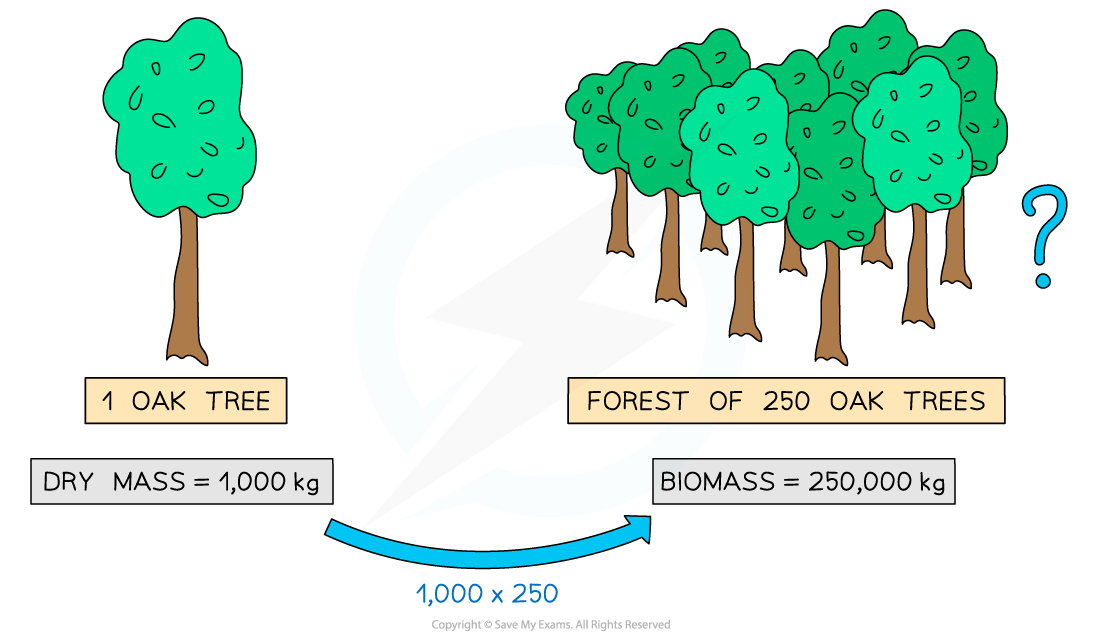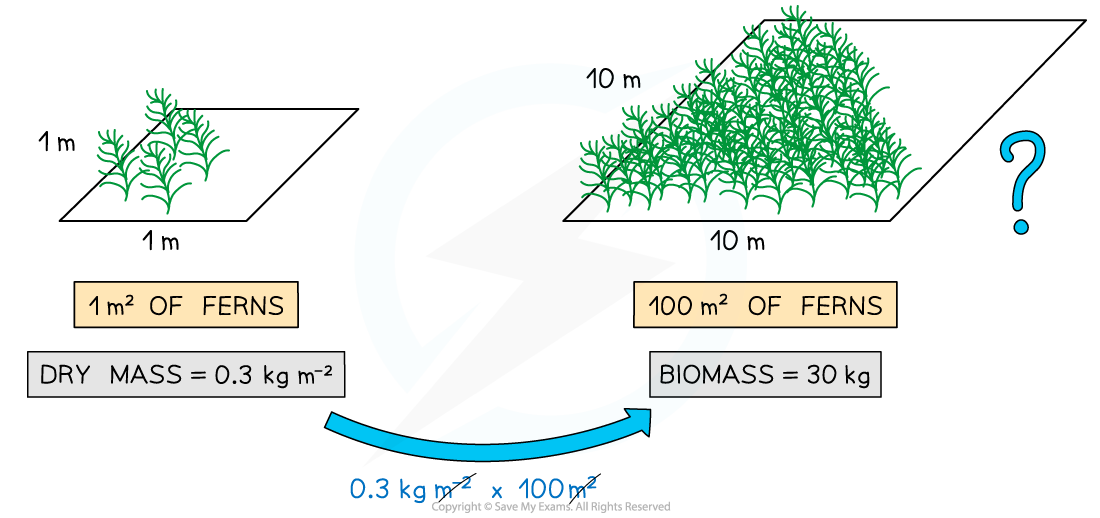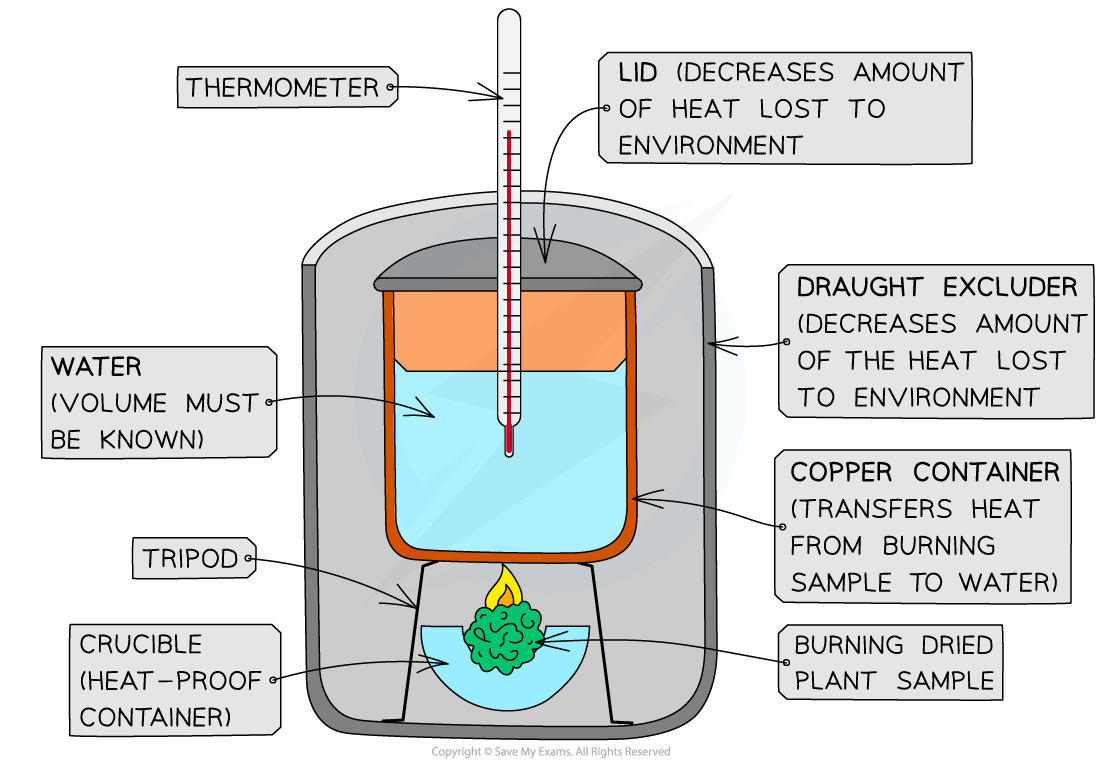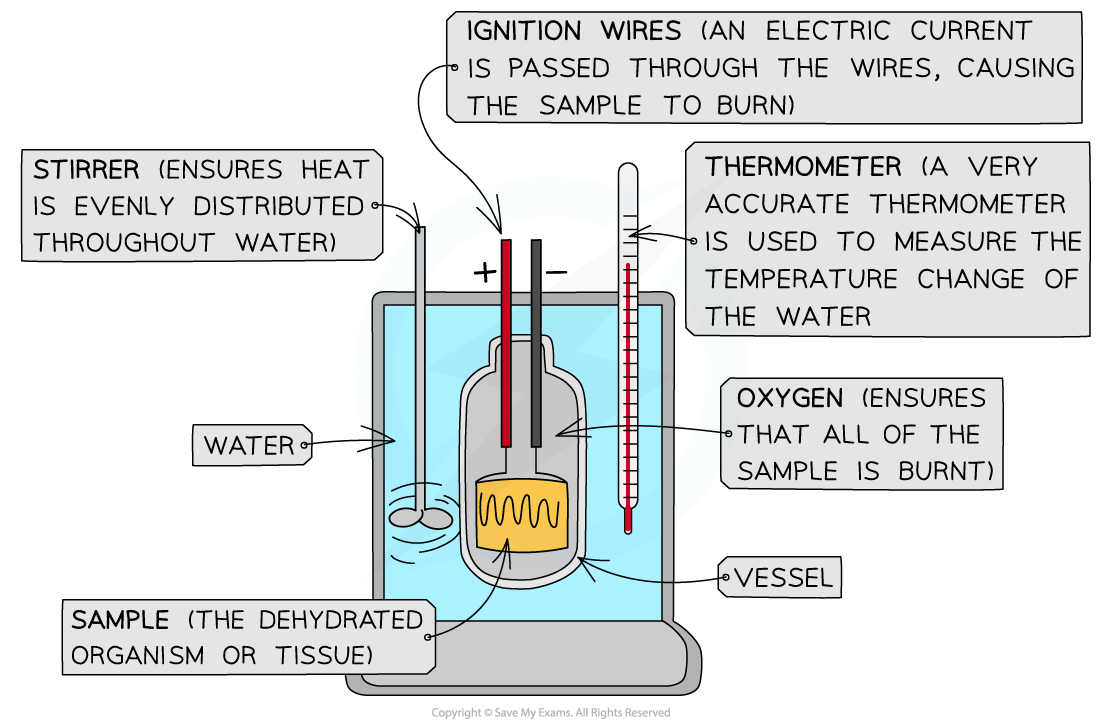# AQA A Level Biology复习笔记5.3.3 Biomass

### Biomass

• The biomass of an organism (or of a sample of tissue from an organism) is:
• The mass of living material of the organism or tissue
• The chemical energy that is stored within the organism or tissue

• Biomass can be measured in terms of:
• The dry mass of an organism or tissue (in a given area)
• The mass of carbon that an organism or tissue contains
• The mass of carbon that a sample (i.e. an organism or tissue) contains is generally taken to be 50% of the dry mass of the sample

#### Dry mass

• The dry mass is the mass of the organism or tissue after all the water has been removed
• The dry mass of a sample can be used to calculate the biomass of a total population of organisms or of a particular area. For example:
• If the dry mass of one daffodil plant is found to be 0.1 kg, then the dry mass (i.e. the biomass) of 200 daffodils would be 20 kg (0.1 x 200 = 20)It is possible to estimate the biomass of a group of organisms if you know the dry mass of a single organism

• If the dry mass of the grass from 1 m² of a field is found to be 0.2 kg, we can say that the grass has a dry mass (i.e. biomass) of 0.2 kg m⁻² (this means 0.2 kg per square metre). If the grass field is 200 m² in size, then the biomass of the whole field must be 40 kg (0.2 x 200 = 40)It is possible to estimate the biomass of organisms in a larger area if you know the dry mass of the organisms in a given (smaller) area

Biomass can change over time

• For example, the biomass of deciduous trees decreases over autumn and winter as they lose their leaves
• This means that biomass is sometimes given with units of time as well
• This shows the average biomass of an organism within a given area over that time period
• For example, if the average biomass of a group of oaks trees over the course of a year is found to be 13,000 kg, the biomass may be given as 13,000 kg y⁻¹ (this means 13,000 kg per year)
• If the average biomass of 1 m² of a grass field over the course of a year is found to be 0.15 kg m⁻², the biomass may be given as 0.15 kg m⁻² yr⁻¹ (this means 0.15 kg per square metre per year)

#### Calorimetry

• Calorimetry can be used to estimate the chemical energy stored in dry biomass
• This involves burning the sample of dry biomass in a piece of equipment known as a calorimeter
• The burning sample heats a known volume of water
• The change in temperature of the water provides an estimate of the chemical energy the sample contains

### Apparatus & Techniques: Finding the Dry Mass and Energy Value of Plant Biomass

• Dry mass is used to measure the mass of living material in a plant sample
• The dry mass is the mass of the organism or tissue after all the water has been removed
• To find the dry mass, the sample must first be dehydrated (dried out until it contains no more water)

• A calorimeter can then be used to estimate the chemical energy stored within the dried plant sample

#### Apparatus

• A heat-proof, open-topped container
• This is often referred to as a crucible
• It needs to be able to withstand the temperatures inside the oven
• It is open-topped to allow any moisture leaving the sample can escape and evaporate

• Oven
• To slowly dry the sample

• Digital balance
• For monitoring the mass of the plant sample as it dries out
• Needs to have a high level of precision to detect small changes in the mass of the sample

• Calorimeter
• These can be simple and inexpensive (easy for students to set up using classroom equipment) or very precise, expensive pieces of apparatus known as bomb calorimeters (more commonly found in professional scientific laboratories)

#### Method

Finding the dry mass of a plant sample:

• Weigh the crucible (heat-proof container) without the sample first
• Place the sample in the crucible
• Place the crucible in the oven
• Set the oven to a low temperature (if the temperature is too high the sample may burn, which would cause it to lose biomass)
• Remove and weigh the crucible (containing the sample) at regular intervals during the drying process
• Once the mass of the crucible (and sample) stops decreasing and becomes constant, the sample is fully dehydrated (all the water has been removed)
• From this final constant mass, subtract the original mass of the crucible (without the sample in it) to find the dry mass of the sample

Finding the energy released by a sample of plant biomass:

• To estimate the chemical energy stored in the dried sample, use a calorimeter
• A calorimeter burns the dried sample and uses the energy released to heat a known volume of water
• Measure the change in temperature of the water
• This temperature change can be used to estimate the chemical energy stored within the sample
• This energy is measured in joules (J) or kilojoules (kJ)
• 1 joule is the amount of heat needed to raise the temperature of 24 g or 0.24 cm3 or 0.24 ml of water by 1 °C
• 1 kilojoule (kJ) is the amount of heat needed to raise the temperature of 240 g or 240 cm3 or 240 ml of water by 1 °C (or to raise the temperature of 24 g of water by 10 °C)A simple, inexpensive version of a calorimeter that can be set up using classroom equipmentAn example of a more precise (and much more expensive) version of a calorimeter known as a bomb calorimeter - this type is used in professional scientific laboratories

#### Limitations

• It can take a long time to fully dehydrate (dry out) a plant sample to find its dry mass
• This is partly because the sample has to be heated at a relatively low temperature to ensure it doesn’t burn
• Depending on the size of the sample, the drying process could take several days

• Precise equipment is needed, which may not be available
• A very precise digital balance should be used to measure the mass of the plant sample as it is drying (to detect even extremely small changes in mass)
• It is preferable to use a very precise digital thermometer when measuring the temperature change of the water in the calorimeter (again, to detect even very small temperature changes)

• The more simple and basic the calorimeter, the less accurate the estimate will be for the chemical energy contained within the plant sample
• This is due to heat energy from the burning sample being lost and not being transferred efficiently to the water
• A bomb calorimeter ensures that almost all the heat energy from the burning sample is transferred to the water, giving a highly accurate estimate